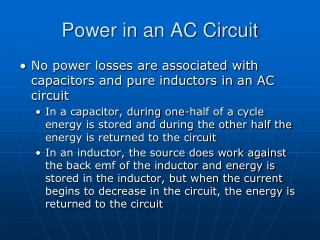DownloadDownload PresentationPower in an AC Circuit

# Power in an AC Circuit

Download Presentation## Power in an AC Circuit

- - - - - - - - - - - - - - - - - - - - - - - - - - - E N D - - - - - - - - - - - - - - - - - - - - - - - - - - -
##### Presentation Transcript

1. Power in an AC Circuit • No power losses are associated with capacitors and pure inductors in an AC circuit • In a capacitor, during one-half of a cycle energy is stored and during the other half the energy is returned to the circuit • In an inductor, the source does work against the back emf of the inductor and energy is stored in the inductor, but when the current begins to decrease in the circuit, the energy is returned to the circuit

2. Power in an AC Circuit, cont • The average power delivered by the generator is converted to internal energy in the resistor • Pav = IVR = IV cos  • cos  is called the power factor of the circuit • Phase shifts can be used to maximize power outputs

3. Resonance in an AC Circuit • Resonance occurs at the frequency, ƒo, where the current has its maximum value • To achieve maximum current, the impedance must have a minimum value • This occurs when XL = XC

4. Resonance, cont • Theoretically, if R = 0 the current would be infinite at resonance • Real circuits always have some resistance • Tuning a radio • A varying capacitor changes the resonance frequency of the tuning circuit in your radio to match the station to be received • Metal Detector • The portal is an inductor, and the frequency is set to a condition with no metal present • When metal is present, it changes the effective inductance, which changes the current which is detected and an alarm sounds

6. Summary of Circuit Elements, Impedance and Phase Angles

7. James Clerk Maxwell • Electricity and magnetism were originally thought to be unrelated • in 1865, James Clerk Maxwell provided a mathematical theory that showed a close relationship between all electric and magnetic phenomena

8. Maxwell’s Starting Points • Electric field lines originate on positive charges and terminate on negative charges • Magnetic field lines always form closed loops – they do not begin or end anywhere • A varying magnetic field induces an emf and hence an electric field (Faraday’s Law) • Magnetic fields are generated by moving charges or currents (Ampère’s Law)

9. Maxwell’s Predictions • Maxwell used these starting points and a corresponding mathematical framework to prove that electric and magnetic fields play symmetric roles in nature • He hypothesized that a changing electric field would produce a magnetic field • Maxwell calculated the speed of light to be 3x108 m/s • He concluded that visible light and all other electromagnetic waves consist of fluctuating electric and magnetic fields, with each varying field inducing the other

10. Hertz’s Confirmation of Maxwell’s Predictions • Heinrich Hertz was the first to generate and detect electromagnetic waves in a laboratory setting

11. James Clerk Maxwell’s Equations (1867) E-field comes out from p-charge and terminates at negative charge. Pre-Maxwell B-field cannot do like E-field. No magnetic monopole! Faraday’s law Ampere’s law

12. The velocity of transverse undulations in our hypothetical medium, calculated from the electromagnetic experiments, agrees so exactly with the velocity of light calculated from the optical experiments, that we can scarcely avoid the inference that light consists in the transverse undulation of same medium which is the cause of electric and magnetic Phenomena. 2.9986 x 108 m/s

13. Hertz’s Experiment (1887)

14. Hertz’s Basic LC Circuit • When the switch is closed, oscillations occur in the current and in the charge on the capacitor • When the capacitor is fully charged, the total energy of the circuit is stored in the electric field of the capacitor • At this time, the current is zero and no energy is stored in the inductor

15. LC Circuit, cont • As the capacitor discharges, the energy stored in the electric field decreases • At the same time, the current increases and the energy stored in the magnetic field increases • When the capacitor is fully discharged, there is no energy stored in its electric field • The current is at a maximum and all the energy is stored in the magnetic field in the inductor • The process repeats in the opposite direction • There is a continuous transfer of energy between the inductor and the capacitor

16. Hertz’s Experimental Apparatus • An induction coil is connected to two large spheres forming a capacitor • Oscillations are initiated by short voltage pulses • The inductor and capacitor form the transmitter

17. Hertz’s Experiment • Several meters away from the transmitter is the receiver • This consisted of a single loop of wire connected to two spheres • It had its own inductance and capacitance • When the resonance frequencies of the transmitter and receiver matched, energy transfer occurred between them

18. Hertz’s Conclusions • Hertz hypothesized the energy transfer was in the form of waves • These are now known to be electromagnetic waves • Hertz confirmed Maxwell’s theory by showing the waves existed and had all the properties of light waves • They had different frequencies and wavelengths